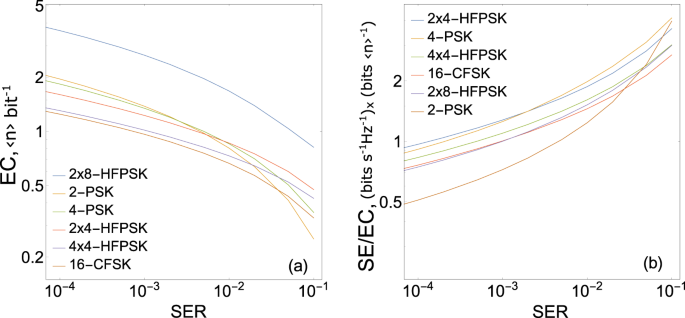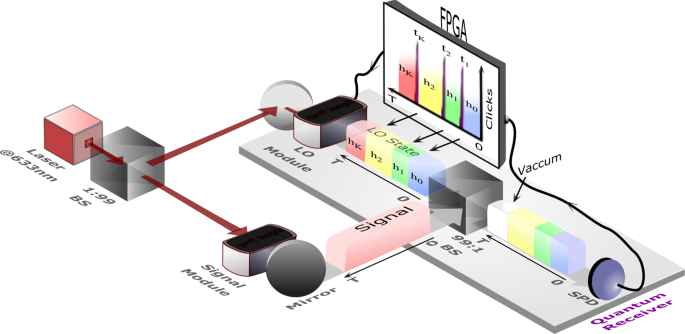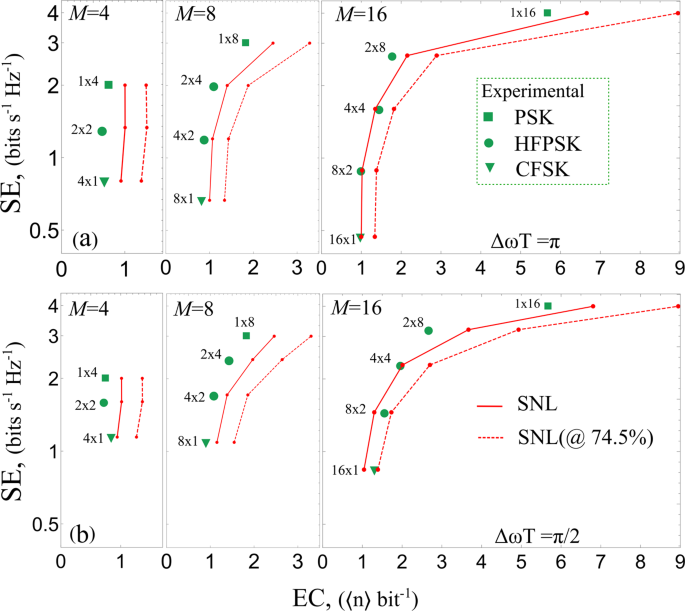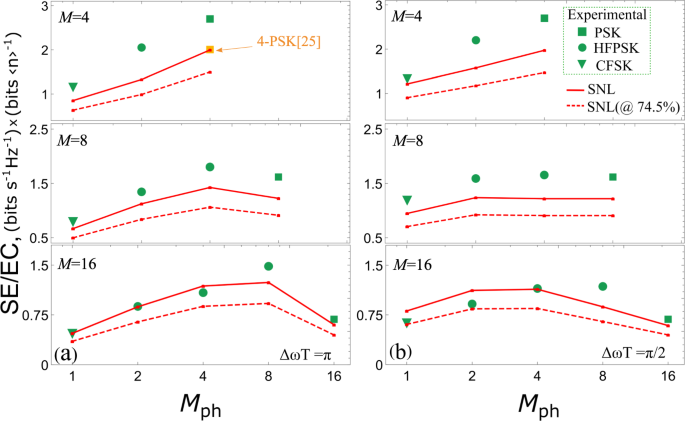## Introduction

Optical communication plays a pivotal role in establishing the global internet. Indeed, optical technologies enable fast, energy-efficient communications compared to electronic technologies. Thanks to optical technologies, broadband Internet became affordable and is now an integral part of life1. Terabits of data are transported through global networks, using nearly all the available capacity of current optical backbone, it is approaching to the so-called “capacity crunch”2. The exponential expansion of the Internet has no sign of slowing down3. To avoid the exponential growth of physical resources required to support this expansion, it is imperative to look for new enabling technologies, such as quantum measurement.

Bandwidth and energy are two fundamental resources needed to exchange information4. In digital communication, information is encoded in a finite set of physical states known as symbols. In optics, modulated laser pulses are often used as information carriers. Indeed, coherent laser light is easy to generate, modulate, and detect even in lossy communication channels5. Modulation schemes differ in their use of parameters such as frequency, phase and/or amplitude of coherent states. The number of states used for data encoding or the alphabet length may also vary. The modulation scheme and the alphabet length are selected to optimize data transfer given the properties of a practical communication channel. However, there is a fundamental limitation on such optimization5,6,7. Even when the classical receiver is ideal and the communication channel is noiseless, communication is limited by shot noise of the measurement. The shot noise limit (SNL) is defined as the minimal possible probability of error also known as symbol error rate (SER) that can be attained by an ideal classical measurement with zero loss and 100% efficient detector, further we will refer to this approximation as to unit system efficiency. Therefore, SNL sets the minimum energy required at the receiver to classically discriminate states with the desired SER. A lower error bound is set by quantum theory, known as the Helstrom bound8.

Much progress has been made to push SER below the SNL and towards the Helstrom bound. The Dolinar receiver uses optimal coherent state displacement9 and can reach the Helstrom bound for the alphabet of two coherent states. Unless the universal quantum computer is available there is no known practical quantum measurement that can reach the Helstrom bound for longer alphabets10. Still, multiple practical methods that can perform below the absolute SNL were proposed11,12,13,14,15,16,17. Most of the quantum receivers considered to date are based on adaptive displacement followed by single photon detection18. A number of experiments demonstrate the quantum advantage over classical receivers with SNL adjusted for loss and non-ideal detector efficiency, i.e. the SER of the ideal classical receiver with the same loss and detection efficiency as in an experiment19,20,21,22,23,24,25,26,27,28,29,30,31,32,33,34. In certain experiments, quantum receivers perform below the absolute SNL, i.e. the SER of the ideal classical receiver with unit system efficiency21,24,25,30,31,32,33,34. Most of the above receivers split the input signal to finite number of copies (or segments) temporally or spatially and measure segments sequentially, adapting the feedback or feedforward at the beginning of each segment. Recently, a quantum receiver with adaptive feedback based on photon detection times, the time-resolving receiver, was experimentally demonstrated33.

Until very recently, quantum measurement-enabled communication systems were studied with modulations developed for classical receivers (legacy classical modulations), such as phase-shift keying (PSK)19,20,21,22,23,24,25,26,27,28,29,30,31 and pulse-position modulation (PPM)14. However, it turns out that the SER values of time-resolving receivers can be further optimized with the help of a specially developed modulation, the coherent frequency shift keying (CFSK)17. Improving the energy efficiency often requires an exponential reduction of the bandwidth efficiency (and vice versa), i.e. energy efficiency can be improved by communication protocols using larger communication channel bandwidth. Here we are interested in practical quantum-enabled communication methods that optimize the use of both resources at the same time.

The notable feature of the time-resolving quantum receiver is that it can be applied to many modulation methods, including PSK and CFSK, by changing the Bayesian likelihoods used by time-resolving adaptive strategy, implemented in the FPGA firmware, only. Using this versatile receiver as a testbed, we present a systematic theoretical and experimental study of the combined resource use by traditional and quantum-inspired modulation schemes for a range alphabet lengths, M = 4–16. The results of this study show that practical quantum measurement can tame the overall resource use in classical communications.

In this work, we introduce a quantum-inspired modulation, specifically designed to optimize both energy and bandwidth efficiency, the hybrid frequency-phase shift keying (HFPSK). We present a unified theoretical framework that describes modulation properties and measurement properties for all communication protocols that were implemented and investigate their theoretical limits. We show theoretically that HFPSK can outperform other encodings in combined resource use.

We experimentally demonstrate SER below the absolute SNL for the HFPSK protocols and identify most efficient encodings among PSK, HFPSK and CFSK protocols with alphabet length from M = 4–16. We show experimentally that HFPSK yields the most dramatic communication channel resource optimization for long communication alphabets of more than 4 symbols. In doing so, we set record energy sensitivities for legacy 4-PSK and 8-PSK, and report the receiver that outperforms the absolute SNL for 16-PSK.

## Results and discussion

### Modulation schemes

In this section we introduce quantum-inspired modulation schemes for optical communication with coherent optical states. We investigate theoretical resource efficiency of these schemes at their Helstrom bounds (HBs) to reveal possible advantage. Lastly, we generalize the discrimination strategy of the time-resolving receiver17,33 for the quantum-inspired modulation schemes.

Consider an M-ary alphabet of rectangular coherent optical pulses with duration T. The alphabet consists of symbols corresponding to M coherent states $$\{\left|\alpha ({\omega }_{i},{\theta }_{ij})\right\rangle \}$$. For each frequency ωi = ω0 + (i − 1)Δω, i 1…Mf the initial phase is θij = (i − 1)Δθf + (j − 1)Δθph where j 1. . . Mph and Δθph = 2π/Mph, such that M = Mf × Mph. Clearly, for each M, there may be several encodings depending on the choice of Mf and Mph. This modulation can be seen as a generalization of the PSK and CFSK schemes because for Mf = 1 it becomes the PSK, and for Mph = 1 it becomes the CFSK. Note that we are particularly interested in small frequency detunings, such that the adjacent frequencies are non-orthogonal: (ωi − ωi−1)T < 2π.

For example, Fig. 1 shows the constellation diagrams of four possible non-orthogonal encoding schemes for the alphabet length of M = 8, which encodes $${\log }_{2}(8)=3$$ bits per symbol. Filled circles represent symbols, whose color represents the frequency and the angular position of a circle represents the phase shift at the beginning of the pulse. The first scheme, top left, is the traditional PSK, where eight symbols are encoded as a phase of one carrier frequency Mf = 1, M = Mph = 8. Second and third encodings correspond to Mf × Mph - HFPSK in which symbols are encoded in two frequencies and four phases, Mf × Mph = 2 × 4, and four frequencies and two phases, Mf × Mph = 4 × 2, respectively. The last one corresponds to CFSK17, where all eight symbols have a different frequency M = Mf = 8, Mph = 1. The constellation diagram for different modulation schemes illustrates that the symbols can be encoded in either phase or frequency, or both phase and frequency.Fig. 1: Constellation diagrams of PSK, CFSK, and HFPSK for M = 8.

### Energy consumption and bandwidth use

To estimate the energy efficiency of these modulation schemes we compare their performance at the fundamental quantum limit - the Helstrom bound (HB)8. The HB relates the energy of the symbol at the input to the lowest possible symbol error rate (SER). For simplicity, we use square root measure to calculate HBs for the above modulation schemes22,35,36,37, which bounds the HB from above. The exact calculation38 yields a more favorable HB for CFSK. However, for signal energies considered in the manuscript (〈n〉 > 0.5) the improvement is negligible (1%). The energy consumption (EC) is defined as an average number of photons per bit (〈n〉  bit−1) required for reliable communication:

$${{{\rm{EC}}}}=\frac{{\left.\langle n\rangle \right|}_{{{{\rm{SER}}}}}}{{\log }_{2}(M)},$$
(1)

where 〈n〉 is the average number of photons per symbol to obtain desired SER. Normalizing the required energy by the number of bits per symbol offers a direct energy requirement comparison between alphabets with different M. In Fig. 2(a) one can see how energy consumption in photons per bit depends on desired SER for different modulation schemes considering the ideal quantum receiver. Clearly, energy consumption increases with M for protocols like PSK even if the receiver operates at the quantum limit. It is not surprising that CFSK provides the lowest energy consumption. Among modulation schemes investigated in this paper, 16-CFSK has the lowest energy consumption. To avoid graph cluttering we do not show all modulation schemes in the figure.Fig. 2: Resource use of modulation schemes vs SER at the HB and their comparison to legacy modulation schemes.

The spectral efficiency (SE), SE $$=\,{\log }_{2}M/B$$, is conventionally defined as information rate (bits  s−1) over unit bandwidth, B (Hz) and unit of measurement is bits  s−1 Hz−1. This definition also allows comparison between the alphabets with different M. The SE for the protocols considered here can be found:

$${{{\rm{SE}}}}=\frac{{\log }_{2}({M}_{{{{\rm{f}}}}}\times {M}_{{{{\rm{ph}}}}})}{1+[({M}_{{{{\rm{f}}}}}-1)\frac{\Delta \omega T}{2\pi }]}.$$
(2)

Clearly, PSK takes the smallest bandwidth. Note that even though CFSK uses more bandwidth than other communication protocols of this family, it is still more spectrally efficient than legacy orthogonal FSK that requires orthogonal states (i.e. ΔωT≥2π), because CFSK detunings are ΔωT < 2π.

The combined resource-efficiency measure can be introduced as SE/EC. In Fig. 2(b) we present the combined resource efficiency for the modulation schemes shown in Fig. 2(a). The potential advantage in the combined resource efficiency of the 2 × 4-HFPSK modulation for SERs smaller than 2 × 10−3 at the HB is seen. This 2 × 4-HFPSK has the highest combined resource efficiency amongst all modulation schemes investigated here.

### The time-resolving quantum receiver

Our quantum receiver schematically depicted in Fig. 3 and identified by the gray-shaded rectangle employs an adaptive displacement followed by a time-resolved single-photon detection. The input signal s = {sf, sph} is displaced with the local oscillator (LO) whose parameters correspond to a hypothesis h = {hf, hph}. A hypothesis h is intended to displace the input signal to the vacuum if h = s. However, any incorrect guess h ≠ s can result in a photon detection. The hypotheses is based on the current knowledge of the maximally likely input $$\mathop{\max }\limits_{m}(\wp (m))$$, m = {mf, mph}. To calculate probabilities $${\wp }_{{t}_{k}}(m)$$ Bayesian inference is used. Initially (at t = 0) all hypotheses have equal probabilities, $$\wp{_{0}} (m)$$ = 1/M. With each detection of a kth photon at time tk, the set of Bayesian probabilities $$\{{\wp }_{{t}_{k}}(m)\}$$ is recalculated, and the new hypothesis hk is updated to the state with the highest a posteriori probability $$\mathop{\max }\nolimits_{m}({\wp }_{{t}_{k}}(m))$$. At the time T, the most likely hypothesis is the received state. Notice that the likelihood to detect a photon strongly depends on detection time tk for CFSK and HFPSK modulations. In turn, posterior Bayesian probabilities, $$\{{\wp }_{{t}_{k}}(m)\}$$, strongly depend on tk. Thus, photon detection times can carry additional information about the input state. See Supplementary Note I for the derivation of Bayesian probabilities and an illustrative example. The distinctive feature of this quantum receiver is that it updates the hypothesis and displaces the signal immediately after each photon detection instead of using predefined measurement stages of fixed duration and a decision-making tree, which was the case in most receivers demonstrated to date. The time-resolving property enables quantum-inspired modulation methods and improves the receiver’s performance for conventional modulation methods, such as PSK. Ideally, our time-resolving receiver can be seen as a multi-stage quantum receiver with the infinite number of measurement stages and the infinite decision-making tree. The practical performance of our receiver is limited by the finite feedback and switching times in the experiment and can be observed as SER saturation for states with the higher average number of photons (see Supplementary Fig. 3).Fig. 3: The experimental implementation of our time resolved quantum receiver testbed.

The performance of the quantum receiver and its dependence on modulation parameters ΔωT and Δθf is assessed through a numerical simulation. Photon detections are simulated using the time-dependent mean photon number, assuming the ideal single photon detector (SPD). The Bayesian algorithm is fed with detection times and the a posteriori hypothesis is computed for every such detection. The received state is compared with the input state and the SER is statistically estimated. The modulation parameter ΔωT is set to π and π/2 and Δθf are chosen via a numerical optimization, see Supplementary Note II for details.

The experimental implementation of our receiver is described in the “Methods” section. To put simulations and experimental results in context, we derived the SNL for the HFPSK protocol family, see Supplementary Note III. To discriminate the input signal state, an arbitrarily chosen initial hypothesis h0 is sent to the beam splitter. The displaced input signal is sent to the SPD.

### Experimental results

Our experimental results show a significant advantage of the quantum measurement over the classical measurement for long (M ≥ 4) communication alphabets. Particularly, we experimentally demonstrate the error rates below the SNL adjusted for system efficiency in all experimental configurations. In addition, the quantum advantage obtained in the experiment was significant enough to reduce SER below the absolute SNL for most of the modulation schemes investigated here. We point out that we report the record EC (at SER = 15%) for the legacy PSK protocol with M = 4, 8, and 16. This work reports below-the absolute SNL EC for the 16-PSK. These significant improvements in PSK measurements are thanks to measurement advantages of the time-resolved quantum receiver. Detailed experimental results are presented in Supplementary Fig. 3 and Supplementary Table 1.

To demonstrate experimental trade-offs in the resource use, we plot the SE vs EC for the fixed SER. Here we chose SER = 15%, because most of the modulation schemes considered here can achieve this SER with EC  1 photon/bit, which is often the goal. While the SE is determined by the encoding scheme parameters only, Eq. (2), the EC, Eq. (1) is measured in the experiment. To estimate the EC for the desired SER, we measure the SER for the input signal in a range of the average number of photons and use linear interpolation in logarithmic scale (see Supplementary Results for details).

We present our experimental data in Fig. 4. We plot SE versus the experimentally measured EC for different alphabet lengths M= 4, 8 and 16. For comparison, we also plot the corresponding SNLs. Note that our SNLs are separately optimized vs. the phase parameter Δθf so that our experiment is fairly compared with the best possible classical receiver with the matched encoding and the same SE. It is evident from the figure that all the experimental data points for shorter (M ≤ 8) alphabets outperform the absolute SNL by the EC. For all alphabet lengths, the experimental SER outperforms the adjusted SNL. As expected, the use of the smaller ΔωT = π/2 detuning gives a nearly twofold advantage in SE whereas using a larger ΔωT = π detuning reduces the EC.Fig. 4: Spectral efficiency (SE) vs. experimentally measured energy consumption (EC) at SER = 15%.

### Discussion

Our experimental demonstration shows, Fig. 4, that the energy per bit requirement for a longer-alphabet PSK modulation rapidly grows with M even when a quantum receiver is used. This behavior is consistent with the exponential growth of the energy requirement with M known from theory. We see that this growth can be significantly tamed with a small expansion into the bandwidth use. Particularly, for M = 16, PSK requires nearly 2.2 times more energy per pulse than the Mf × Mph = 2 × 8 HFPSK, while the required bandwidth increases by just 1.3 times, Fig. 4(b). Similarly, the CFSK requires the lowest energy for the same error rate, but it expands into bandwidth space (even though it does so to a lesser extent than classical bandwidth-limited encodings). At M = 16, the use of 8 × 2-HFPSK can reduce the bandwidth nearly by the factor of two, while the required energy increases by less than 2% in comparison to 16-CFSK, as seen in Fig. 4(a). For a shorter alphabet, M = 4, the trade-off between EC and SE is less pronounced, as can be seen from our experimental results. Still, for a channel with significant energy limitations the HFPSK modulation is the best candidate. Thus, the flexibility offered by the HFPSK provides a significant optimization space for communication channels with bandwidth and/or energy restrictions. The optimal encoding choice is therefore tied to available channel resources.

For example, if both SE and EC are equally important, the combined resource efficiency is SE/EC. In Fig. 5 we plot experimentally measured resource efficiencies compared to the SNL for the same protocols as in Fig. 4. Here, Δθf is also optimized separately for our receiver and for the SNL calculation to achieve a fair comparison. Thus, even when HFPSK is specifically optimized for the ideal classical measurement, the classical measurement cannot offer a better SE/EC than what can be achieved with our quantum measurement scheme. It can be seen that the HFPSK has the highest resource efficiency for longer alphabet lengths (M = 8 and M = 16) for protocols with the same M. Note that this HFPSK advantage is evident even without reaching the exact minimum of the SE/EC for HFPSK. A significant improvement in the resource efficiency of time-resolving receiver can be seen in comparison to the previous best known 4-PSK experimental result (yellow filled square) extracted from ref. 25 for SER = 15%.Fig. 5: Combined resource efficiency at SER = 15% plotted against Mph for different alphabet lengths M.

In summary, we have implemented a versatile software-reconfigurable quantum receiver platform and demonstrated quantum measurement advantage for a range of modulations and alphabet lengths (up to M = 16). We demonstrated experimental SER below the ideal SNL for the legacy 16-PSK protocol. We introduced and experimentally verified the below SNL error rates of all M-ary HFPSK modulation schemes. This systematic study of quantum receivers and quantum-enabled modulation methods provides a better understanding of a trade-off between SE and EC, thus enabling a quantum-enhanced optical communication channel optimization. The modulation method introduced here, the HFPSK, not only gives an extra flexibility for such optimization, but theoretically allows better resource efficiency than any other modulation scheme. Surprisingly, Mf × Mph = 2 × 2 HFPSK yields the lowest EC in the experiment at SER=15%. We also show experimentally that the HFPSK yields the best resource optimization for longer alphabets (i.e. M > 4). We expect that this trend continues for even longer alphabet lengths. We point out that although the quantum-inspired modulation schemes, such as CFSK and HFPSK, can in principle be used in conjunction with a classical receiver, the design of an efficient classical receiver can be significantly more complex than that of a receiver for legacy PSK. In contrast, as shown in this paper, the hardware design of a quantum receiver is identical for both legacy and quantum-inspired modulation schemes. The only difference between the quantum receivers for different modulations considered here is the Bayesian likelihood used by the adaptive strategy, implemented in the FPGA firmware. The results of this work can be used for further development of quantum-enabled optical communication systems, and for better understanding of properties of the quantum measurement.

## Methods

### Experimental implementation of the time-resolving quantum receiver

The experimental setup closely follows the conceptual diagram in Fig. 3. A laser at 633 nm is sent to a 1:99 beam splitter and then transmitted and reflected beams are sent to a signal preparation module and an LO preparation module, respectively. Signal and LO preparation modules, shown as black boxes, are comprised of the double-pass acousto-optic modulator (AOM)39. The quantum receiver, highlighted with a shaded rectangle, is comprised of an LO preparation module, a 99:1 beamsplitter, an single-photon detector (SPD) and a field programmable gate array (FPGA). The signal preparation module generates a desired input signal state. The LO preparation module generates the optical state corresponding to the current hypothesis h. Encoding methods are changed by reprogramming the FPGA and no change in the physical layout is needed. This versatility of the experimental setup comes at the cost of long switching time of AOMs of about 1 μs due to a finite speed of sound in the modulator. The frequency shifts are set to the same value of Δω = 2π 7629 rad  s−1 for all modulation schemes tested in this work. To change the dimensionless detuning parameter ΔωT the pulse duration T is adjusted. The system efficiency of the receiver is calculated by measuring optical transmission loss (11.4(5)%) in the optical components and detection efficiency (84.0(3)%) of the SPD. Thus the estimated system efficiency is 74.5(6)%. This system efficiency is used to calculate the adjusted SNL.

The FPGA computes Bayesian probabilities, updates the most likely hypothesis, and switches the LO to the next hypothesis, hk, based on the previous hypothesis hk−1 and detection time tk. The LO change is represented in Fig. 3 by filling color of the LO pulse h0, h1, h2hK, where K is the number of single-photon detections during each measurement. In an experiment the number K randomly changes from one discrimination measurement to the other because of the random nature of the photon detection from a coherent state. This random process is governed by the Poisson distribution. Note that for CFSK and HPFSK the displaced input to the detector may be modulated, thus the posterior hypothesis depends on the exact photon detection time. Therefore, even an ideal receiver tests a different number of hypotheses in each individual measurement before finding the correct displacement, and the correct hypothesis is not always found. At the end of the pulse, i.e. at time T, the current most likely hypothesis hK is our best guess of the input state. If hK matches the signal s = hK, a discrimination is considered successful, otherwise an error is registered. The number of errors is measured and the probability of error is experimentally obtained for a range of input symbol energies. Every time a photon is detected, an electronic pulse is sent to the FPGA. The k-th pulse gets registered at time tk, as shown in the inset of Fig. 3.